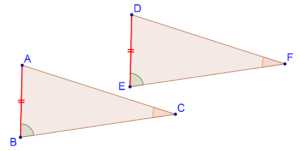# Is There an AAS Criterion?

Is There an AAS Criterion?

We know ASA congruence criterion works when two angles and the included side (the side between the two angles) of one triangle are (correspondingly) equal to two angles and the included side of another triangle. Refer Proof of ASA congruence criterion to understand it better.Think: But will the ASA criterion hold if the equal sides are non-included? In other words, is there something like an AAS criterion?

The answer, as you may be able to anticipate, is YES! If two angles of a triangle are equal to two angles of another, then their third angles are automatically equal, since the sum of angles in any triangle must be a constant ($$180^\circ$$).

## Why AAS congruence criterion works?

Consider the following figure:In $$\Delta ABC$$ and $$\Delta DEF$$, we are given that

1. $$\angle B = \angle E$$

2. $$\angle C = \angle F$$

3. $$AB = DE$$ (a non-included side)

Can we say that the two triangles are congruent? YES, because $$\angle A$$ and $$\angle D$$ must also be equal, and thus our ASA congruence criterion applies as we discussed earlier.

Note: To summarize: when we have to apply the ASA congruence criterion between two triangles, we only need to check that:

1. Two pairs of angles should be (correspondingly) equal.

2. Any pair of sides should be (correspondingly) equal.

grade 9 | Questions Set 2
grade 9 | Questions Set 1
More Important Topics
Numbers
Algebra
Geometry
Measurement
Money
Data
Trigonometry
Calculus
More Important Topics
Numbers
Algebra
Geometry
Measurement
Money
Data
Trigonometry
Calculus